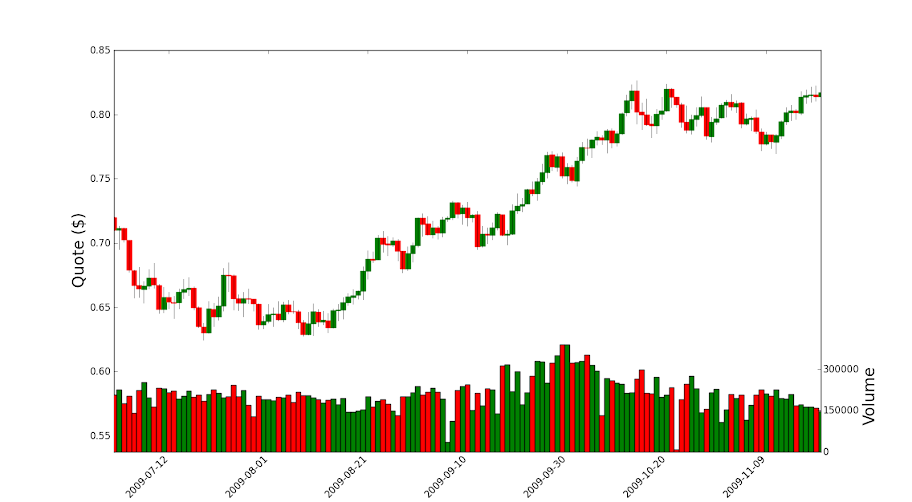# Matplotlib - Finance volume overlay

### Question

I'm making a candlestick chart with two data sets: [open, high, low, close] and volume. I'm trying to overlay the volumes at the bottom of the chart like this:I'm calling volume_overlay3 but instead of bars it fills the whole plot area. What am I doing wrong?My other option is to use .bar(), which doesn't have the up and down colors but would work if I could get the scale right:``````fig = plt.figure()

candlestick(ax, candlesticks)

ax2 = ax.twinx()

volume_overlay3(ax2, quotes)

ax2.xaxis_date()

ax2.set_xlim(candlesticks, candlesticks[-1])

ax.yaxis.set_label_position("right")
ax.yaxis.tick_right()

ax2.yaxis.set_label_position("left")
ax2.yaxis.tick_left()
``````
1
14
10/29/2012 7:54:17 PM

The volume_overlay3 did not work for me. So I tried your idea to add a bar plot to the candlestick plot.

After creating a twin axis for the volume re-position this axis (make it short) and modify the range of the candlestick y-data to avoid collisions.

``````import numpy as np
import matplotlib
import matplotlib.pyplot as plt
# from matplotlib.finance import candlestick
# from matplotlib.finance import volume_overlay3
# finance module is no longer part of matplotlib
# see: https://github.com/matplotlib/mpl_finance
from mpl_finance import candlestick_ochl as candlestick
from mpl_finance import volume_overlay3
from matplotlib.dates import num2date
from matplotlib.dates import date2num
import matplotlib.mlab as mlab
import datetime

datafile = 'data.csv'
r = mlab.csv2rec(datafile, delimiter=';')

# the dates in my example file-set are very sparse (and annoying) change the dates to be sequential
for i in range(len(r)-1):
r['date'][i+1] = r['date'][i] + datetime.timedelta(days=1)

candlesticks = zip(date2num(r['date']),r['open'],r['close'],r['max'],r['min'],r['volume'])

fig = plt.figure()

ax.set_ylabel('Quote (\$)', size=20)
candlestick(ax, candlesticks,width=1,colorup='g', colordown='r')

# shift y-limits of the candlestick plot so that there is space at the bottom for the volume bar chart
yl = ax.get_ylim()

# create the second axis for the volume bar-plot
ax2 = ax.twinx()

# set the position of ax2 so that it is short (y2=0.32) but otherwise the same size as ax
ax2.set_position(matplotlib.transforms.Bbox([[0.125,0.1],[0.9,0.32]]))

# get data from candlesticks for a bar plot
dates = [x for x in candlesticks]
dates = np.asarray(dates)
volume = [x for x in candlesticks]
volume = np.asarray(volume)

# make bar plots and color differently depending on up/down for the day
pos = r['open']-r['close']<0
neg = r['open']-r['close']>0
ax2.bar(dates[pos],volume[pos],color='green',width=1,align='center')
ax2.bar(dates[neg],volume[neg],color='red',width=1,align='center')

#scale the x-axis tight
ax2.set_xlim(min(dates),max(dates))
# the y-ticks for the bar were too dense, keep only every third one
yticks = ax2.get_yticks()
ax2.set_yticks(yticks[::3])

ax2.yaxis.set_label_position("right")
ax2.set_ylabel('Volume', size=20)

# format the x-ticks with a human-readable date.
xt = ax.get_xticks()
new_xticks = [datetime.date.isoformat(num2date(d)) for d in xt]
ax.set_xticklabels(new_xticks,rotation=45, horizontalalignment='right')

plt.ion()
plt.show()
``````data.csv is up here: http://pastebin.com/5dwzUM6e

35
12/4/2017 2:33:48 AM

See the answer here. Apparently a bug and it's going to be fixed.

For now you need to assign the returned collection from the `volume_overlay3` call to a variable then add that to the chart.

``````vc = volume_overlay3(ax2, quotes)